•CODE
• MATLAB实现高斯曲线拟合 完成某次课程任务，在MATLAB中新建一个m文件，将下列代码复制，或者直接复制到命令栏回车即可使用 x=[0:0.1:10];%0—10每次加0.1，共101个点 y=50*exp(-(x-5).^2./2);%x，y为初始函数值表现...
MATLAB实现高斯曲线拟合
完成某次课程任务，在MATLAB中新建一个m文件，将下列代码复制，或者直接复制到命令栏回车即可使用
x=[0:0.1:10];%0—10每次加0.1，共101个点
y=50*exp(-(x-5).^2./2);%x，y为初始函数值表现为红线
x=x';%因为拟合曲线时需要列向量
y=y';
y0=1*randn(1,101);%添加噪声//形成1行101列随机数
y0=y0';%y1为添加噪声后的函数，表现为点
y1=y+y0;%加上噪声后的函数值
Mytype=fittype('A*exp(-(x-u)^2/(2*d^2))');%需要拟合的函数类型
[cf ,gof]=fit(x(:),y1(:),Mytype)%fit函数
xi=0:0.1:10;
yi=cf.A*exp(-(xi-cf.u).^2/(2*cf.d^2));%xi，yi为拟合后的函数
plot(x,y1,'g.');%加噪声的函数为绿色的点
hold on;
plot(xi,yi,'r');%拟合后的曲线为红色的线
plot(x,y,'b');%拟合前的标准曲线为蓝色的线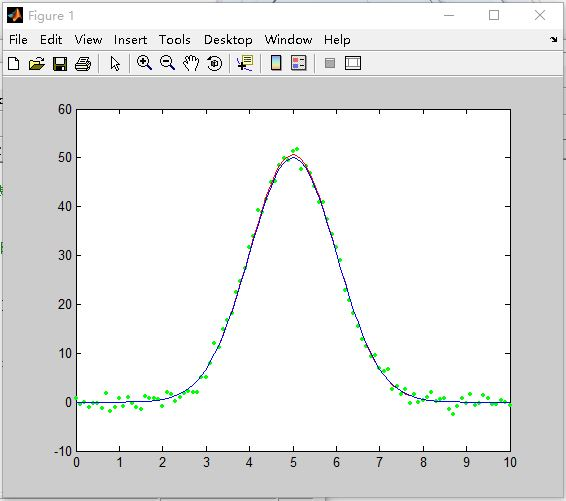展开全文• 多次拟合任意函数曲线，采用高斯曲线拟合算法求解拟合系数。
• https://blog.csdn.net/KEY_Init/article/details/103258920 Matlab进行高斯拟合
https://blog.csdn.net/KEY_Init/article/details/103258920
Matlab进行高斯拟合
展开全文• 最小二乘法高斯曲线拟合，基于最小二乘法拟合高斯曲线，(Least squares Gaussian curve fitting )
• 然后程序尝试使用 MatLab 函数“lsqcurvefit”拟合数据，以找到二维高斯的位置、方向和宽度。 不输入参数执行“mainD2GaussFitRot.m”。 二维高斯函数由函数“D2GaussFunctionRot.m”和“D2GaussFunction.m”定义
• 随着新型冠状病毒COVID-19的威胁遍及世界，我们生活在一个日益担忧的时代，本文用matlab分析COVID-19数据集。
原文链接：http://tecdat.cn/?p=19211
原文出处：拓端数据部落公众号

随着新型冠状病毒COVID-19的威胁遍及世界，我们生活在一个日益担忧的时代，本文用matlab分析COVID-19数据集。
COVID-19数据源
我们检查解压缩的文件。包含:
data.csv -2020年各省/州的全球病例每日水平数据confirmed.csv-确诊病例的时间序列数据deaths.csv-死亡人数的时间序列数据recovered.csv-康复人数的时间序列数据
地图上可视化
我们在地图上可视化已确诊病例的数量。我们首先加载纬度和经度变量。
opts = detectImportOptions(filenames(4), "TextType","string");

数据集包含“省/州”变量，但我们要在“国家/地区”等级汇总数据。在此之前，我们需要稍微整理一下数据。
times_conf.("Country/Region")(times_conf.("Country/Region") == "China") = "Mainland China";
times_conf.("Country/Region")(times_conf.("Country/Region") == "Czechia") = "Czech Republic";

现在，我们可以使用  groupsummary  将已确认的案例相加并平均经纬度来按国家/地区汇总数据。
country = groupsummary(times_conf,"Country/Region",{'sum','mean'},vars(3:end));

输出中包含不必要的列，例如纬度和经度的总和。我们删除这些变量。

vars = regexprep(vars,"^(sum_)(?=L(a|o))","remove_");
vars = regexprep(vars,"^(mean_)(?=[0-9])","remove_");


times_conf_exChina = times_conf_country(times_conf_country.("Country/Region") ~= "Mainland China",:);

让我们使用  geobubble  可视化数据集中的第一个和最后一个日期数据。

for ii = [4, length(vars)]
times_conf_exChina.Category = categorical(repmat("<100",height(times_conf_exChina),1));
times_conf_exChina.Category(table2array(times_conf_exChina(:,ii)) >= 100) = ">=100";
gb.LegendVisible = "off";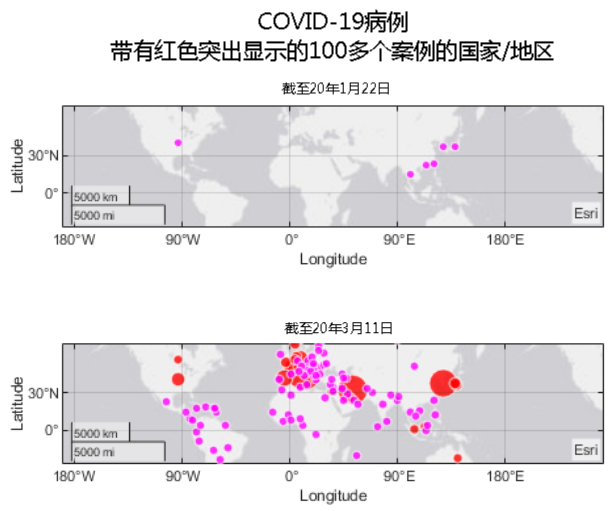我们可以看到它最初只影响了大陆周围的国家/地区。值得注意的是，我们早在2020年1月22日就已经在美国确认了病例。
美国确诊病例
进入美国的省/州级别。
figure
t = tiledlayout("flow");
for ii = [5, length(vars)]

gb.BubbleColorList = [1,0,1;1,0,0];
gb.LegendVisible = "off";
gb.Title = "As of " + vars(ii);
gb.SizeLimits = [0, max(times_conf_us.(vars{length(vars)}))];
gb.MapCenter = [44.9669 -113.6201];
gb.ZoomLevel = 1.7678;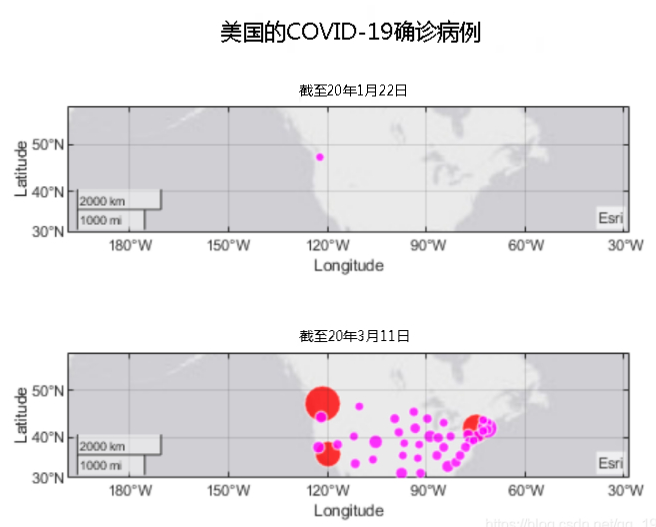可以看到它始于华盛顿，并在加利福尼亚和纽约爆发了大规模疫情。
按确诊病例排名国家/地区
让我们使用covid_19_data.csv比较按国家/地区确认的病例数。日期时间格式中存在不一致之处，因此我们一开始会将其视为文本。
opts = detectImportOptions(filenames(3), "TextType","string","DatetimeType","text");

清理日期时间格式。
Data.nDate = regexprep(Data.Date,"\/20\$","/2020");
Data.Date = datetime(Data.Date);

我们还需要标准化“国家/地区”中的值。
Country_Region(Country_Region == "Iran (Islamic Republic of)") = "Iran";

数据集包含省/州变量。让我们在“国家/地区”级别汇总数据。
countryData = groupsummary(provData,{'ObservationDate','Country_Region'}, ...
"sum",{'Confirmed','Deaths','Recovered'});

countryData包含每日累积数据。我们只需要最新的数字。

确认病例按国家/地区的增长
我们还可以检查这些国家中病例的增长速度。
figure
plot(countryData.ObservationDate(countryData.Country_Region == labelsK(2)), ...
hold on
for ii = 3:length(labelsK)
plot(countryData.ObservationDate(countryData.Country_Region == labelsK(ii)), ...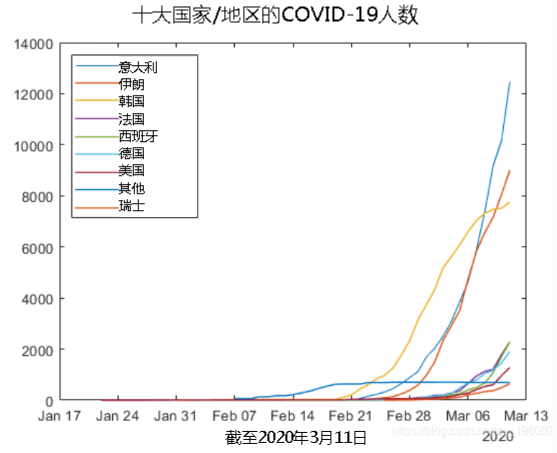尽管韩国显示出增长放缓的迹象，但它在其他地方正在加速发展。
按国家/地区划分的新病例增长
我们可以通过减去两个日期之间已确认病例的累计数量来计算新病例的数量。

for ii = 1:length(labelsK)
country = provData(provData.Country_Region == labelsK(ii),:);
country = groupsummary(country,{'ObservationDate','Country_Region'}, ...

if labelsK(ii) ~= "Others"
nexttile


您可以看到，中国和韩国没有很多新病例。可见，已经遏制住了疫情。
中国
由于中国的感染速度正在放缓，我们来看看仍有多少活跃病例。您可以通过从确诊病例中减去恢复病例和死亡来计算活跃病例。
for ii = 1:length(labelsK)
by_country{ii}.Active = by_country{ii}.Confirmed - by_country{ii}.Deaths -

figure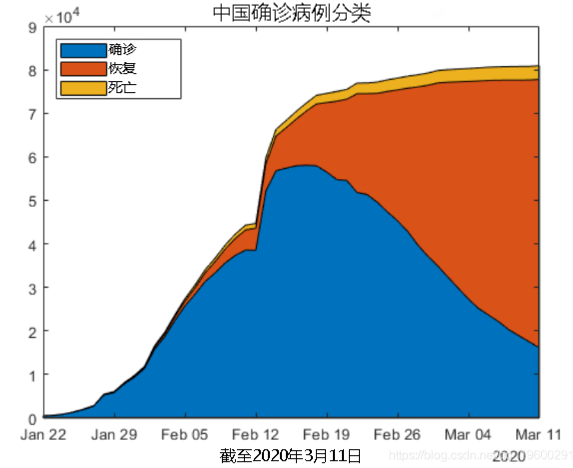拟合曲线
有效案例的数量正在下降，曲线看起来大致为高斯曲线。我们可以拟合高斯模型并预测活动案例何时为零吗？
我使用  曲线拟合工具箱  进行高斯拟合。

ft = fittype("gauss1");

[fobj, gof] = fit(x,y,ft,opts);
gof

gof =
struct with fields:

sse: 4.4145e+08
rsquare: 0.9743
dfe: 47
rmse: 3.0647e+03

让我们通过增加20天来将输出预测。
现在我们对结果进行绘制。
figure
area(ObservationDate,by_country{1}.Active)
hold on
plot(xdates,yhat,"lineWidth",2)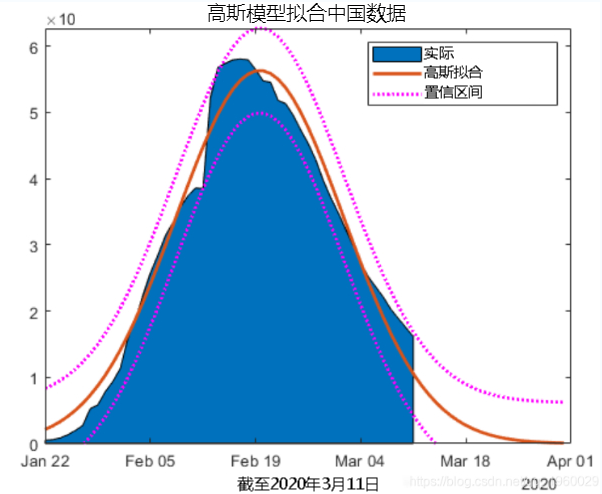韩国
让我们来查看韩国的活跃病例，恢复案例和死亡人数。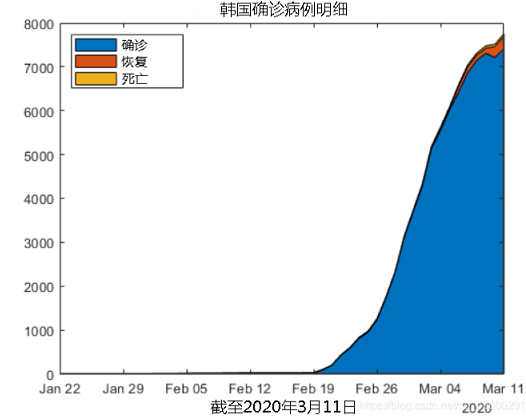使用高斯模型无法获得任何合适的结果。最受欢迎的见解
1.在python中使用lstm和pytorch进行时间序列预测
2.python中利用长短期记忆模型lstm进行时间序列预测分析
3.使用r语言进行时间序列（arima，指数平滑）分析
4.r语言多元copula-garch-模型时间序列预测
5.r语言copulas和金融时间序列案例
6.使用r语言随机波动模型sv处理时间序列中的随机波动
7.r语言时间序列tar阈值自回归模型
8.r语言k-shape时间序列聚类方法对股票价格时间序列聚类
9.python3用arima模型进行时间序列预测
展开全文数据
• FITGAUSS 是通过 Marquardt-Levenberg 非线性最小二乘法最小化将高斯曲线“f”拟合到实验数据的函数。 拟合函数的形式为 a*exp(-((xb)/c)^2)+d*x+e。 这意味着曲线由一条直线和一个高斯曲线构成。 输入：“x,y”是...
• [sigma,mu,A]=mygaussfit(x,y) [sigma,mu,A]=... 拟合是由 polyfit 完成的数据的局域网。 h 是阈值，它是分数从数据的最大 y 高度是从。 h 应该是 0-1 之间的数字。 如果 h 尚未被采用，则将其设置为 0.2 作为默认值。
• 此函数采用一维略带噪声的测试信号，并使用 fminsearch() 函数拟合 6 个高斯函数。 确定每个高斯的参数（幅度、峰值位置和宽度）。 6 个高斯信号应加在一起以给出原始测试信号的最佳估计值。 您可以指定任意数量的...
• 本代码主要利用MATLAB工具实现MATLAB——绘制正态拟合曲线直方图，简单明了，易于理解
• 函数功能：高斯牛顿法实现非线性数据拟合。 代码 clear;clc; M=1000; Te=20; % 模型 t=Te*(1:M)'; load noise; % 噪音可自己添加 Et1=0.4*exp(-t/50)+0.6*exp(-t/200); Et2=0.4*exp(-(t/50).^2)+0.6*exp(-t/200); ...
函数功能：高斯牛顿法实现非线性数据拟合。
代码
clear;clc;
M=1000;
Te=20;
% 模型
t=Te*(1:M)';
Et1=0.4*exp(-t/50)+0.6*exp(-t/200);
Et2=0.4*exp(-(t/50).^2)+0.6*exp(-t/200);
Et3=0.3*exp(-t/50)+0.4*exp(-t/200)+0.3*exp(-t/800);
Et4=0.3*exp(-(t/50).^2)+0.4*exp(-t/200)+0.3*exp(-t/800);
Et = Et4 + noise;
%%
% 选择合适的初始值很重要
% p0=[0.5,10,0.5,100];
p0=[0.5,50,0.5,100,0.5,500]';
% 迭代过程
for i=1:M
[J,dF] = GNA(p0,t,Et);
p = p0+(J'*J)\J'*dF;
if norm(p-p0)/norm(p0)<1e-6
break;
end
p0 = p;
end
disp(p);
fit = p(1)*exp(-(t/p(2)).^2) + p(3)*exp(-t/p(4)) + p(5)*exp(-t/p(6));
plot(t,Et,t,fit)
xlabel('Measured time(\mus)');
ylabel('Amplitude');
title('Models VS Fitting');
legend('Model','Fitting')

function [J,dE]=GNA(p,t,Et)
% 计算Jacobi矩阵和拟合误差
% p为未知参数
p1 = p(1);
p2 = p(2);
p3 = p(3);
p4 = p(4);
p5 = p(5);

p6 = p(6);
f = p1*exp(-(t/p2).^2) + p3*exp(-t/p4) + p5*exp(-t/p6);  % 拟合函数表达式
fp1 = exp(-t.^2/p2^2);
fp2 = (2*p1*t.^2.*exp(-t.^2/p2^2))/p2^3;
fp3 = exp(-t/p4);
fp4 = (p3*t.*exp(-t/p4))/p4^2;
fp5 = exp(-t/p6);
fp6 = (p5*t.*exp(-t/p6))/p6^2;
J = [fp1,fp2,fp3,fp4,fp5,fp6];
dE = Et - f;
计算结果如下：0.3030   48.8801    0.3971  198.6767    0.3057  791.1436；
仅七次迭代就得到了结果，速度快，精度高。
对比Matlab自带函数nlinfit和lsqcurvefit的计算结果：
Nlinfit：0.3030   48.8801    0.3971  198.6768    0.3057  791.1437
Lsqcurvefit：0.3030   48.8795    0.3970  198.6615    0.3057  791.1156

展开全文• 而化学分析中，有许多具有明确物理意义的二维谱图，图光谱、色谱等，其信号峰位置、峰高、峰宽等均具有实际物理意义，因此，提出使用高斯曲线进行数据拟合和表征。 高斯函数仅适用于对称谱图，其简图如下： 假设...信号分析
• 1. 最小二乘法 ...最小二乘法可用于曲线拟合。最小二乘法 线性最小二乘的基本公式 考虑超定方程组（未知数小于方程个数）： 其中m代表有m个等式，n代表有 n 个未知数。方程组满足：m>n 方程组的向...最小二乘法 曲线拟合
• 这是一个用Matlab曲线拟合，预测人口发展。是一个m文件！
• 高斯曲线拟合到数据点。 您可以指定振幅、平均值和标准偏差的初始值，否则它会猜测它们。
• 进行数据点的高斯分布参数拟合之前，有以下几点需要注意： （1） 数据点要近似是高斯分布的形态，即近似符合正太分布的规律； （2）数据集在使用时要进行归一化处理； （3）数据集索引，即数据点的个数要为奇数；...
• 怎么将一个二维高斯转换为两个一维高斯matlab怎么实现
• ## matlab曲线拟合

万次阅读 多人点赞 2015-11-25 22:57:49
曲线拟合不要求逼近函数通过各采样点，但要求尽量的接近这些点，使误差在某种意义上达到最小。 曲线拟合的实现： 在matlab中，用polyfit函数来求得最小二乘拟合多项式的系数，再用polyval函数按所得的多项式计算所...
• matlab应用，是关于cftool工具箱的详细介绍。在数据处理的过程中使用cftool工具箱极为方便
• 文章目录法1：多元非线性回归方法法2：cftool拟合工具箱 法1：多元非线性回归方法 scatter(unnamed(:,1),unnamed(:,2),10,'r','filled') myfunc = inline('beta(1).*sign(x)+beta(2).*x','beta','x'); beta0=[0.5,...
• 本文通过实例对MATLAB曲线拟合工具箱进行详细讲解，帮助大家更容易理解曲线拟合工具箱（cftool）。 目录1.实例介绍2. 进入系统辨识工具箱界面3. 加载数据4. 加载数据5. 选择拟合曲线的类型 1.实例介绍 已知 x = [0 ...
• 高斯多峰拟合，需要数据基本符合高斯分布（正态分布）
• Matlab是一个很强大的数据处理软件，是人们进行数据分析的得力助手。...当需要研究两个变量之间的关系时，经常要用到曲线拟合曲线拟合不仅能给出拟合后的关系式，还能用图形直观的展现出变量之间的关系。 其实用m...
• 通过 matlab 命令行 cftool 调出拟合工具箱，可以对存储在矩阵、数组中的变量群体进行多种数学方式的拟合，导出数据公式供以... 其实用matlab曲线拟合很便捷，下面将以两个变量(y=f(x))为例详细介绍： 1、运行Matl大数据
• % 首先使用Matlab内置的最小二乘函数 polyfit 进行多项式拟合，具体自己查help % 注：type函数可帮助查看源代码 line1 = polyfit(t_g,p_g,1); t_g2 = 1:0.1:5; p_g2 = polyval(line1,t_g2); plot(t_g,p_g,'o',t_g2,p...
• 使用Matlab的cftool工具箱拟合曲线时，发现曲线拟合得效果很好，但把解析表达式取出来进行绘制时，发现误差非常大。通过查资料才得知，因为cftool输出的系数只保留了4位有效数字，才导致了这种情况。所以要如何获得...cftool...

# matlab高斯曲线拟合matlab 订阅